# Peter-Weyl theorem

(Redirected from Peter–Weyl theorem)
Jump to: navigation, search

A theorem on the approximation of functions on a compact topological group by means of representation functions (cf. Representation function). Letrun through a familyof representatives of all equivalence classes for the irreducible continuous unitary representations of a compact group(cf. Representation of a topological group). Let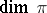be the dimension of a representationand let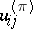be its matrix elements in some orthonormal basis. The Peter–Weyl theorem asserts that the functions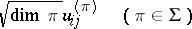form an orthonormal basis in the space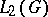of square-summable functions with respect to the Haar measure on(the measure of the entire group is taken to be 1). The algebra of all complex-valued representation functions on, which coincides with the set of finite linear combinations of the functions,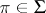, is uniformly dense in the space of all continuous complex-valued functions in.

If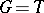is the rotation group for the plane, this assertion coincides with an elementary theorem on approximating periodic continuous functions by trigonometric polynomials.

A consequence of the Peter–Weyl theorem is that the set of linear combinations of characters of the irreducible representations ofis dense in the algebra of all continuous functions on, constant on classes of conjugate elements. Another consequence is that for any element,, there is an irreducible continuous representationofsuch that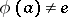; if, on the other hand,is a compact Lie group, thenhas a faithful linear representation.

The Peter–Weyl theorem implies also the following more general assertion , . Suppose one is given a continuous linear representationof a compact groupin a Fréchet space. Then the subspace of representation elements ofis dense in. Here an element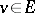is called a representation, or spherical or almost-invariant, element if the orbit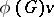generates a finite-dimensional subspace in. This is applicable in particular to the case whereis the space of sections of a certain smoothness class of smooth vector-fibrations, for example, the space of tensor fields of a certain type or given smoothness class on a smooth manifold with a smooth action of a compact Lie group.

The Peter–Weyl theorem was proved in 1927 by F. Peter and H. Weyl .